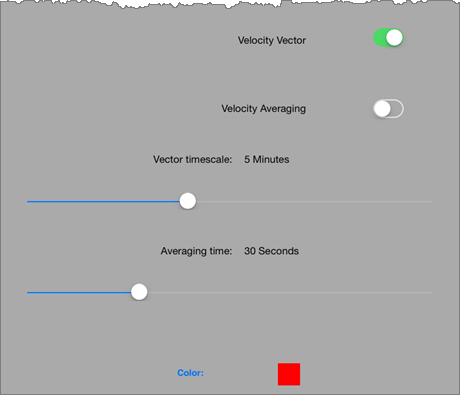# Velocity Vector - What is it?

The line is drawn in the direction you are travelling. The length of the line is designed to show you where you will be within a certain time.

The time shown by the Velocity Vector is configurable from the Settings page.

There are a number of settings on the Settings - GPS Settings page which relate to the Velocity Vector.### Velocity Vector

Turn the Vector Line on or off. The Vector Line is drawn in the direction of travel, and the end of the Vector Line shows where you will be in the time set by the Vector Timescale slider.

### Velocity Averaging

Turn Velocity Averaging on or off. Velocity Averaging smooths out small variations in the calculation of the Velocity Vector caused by intermittent GPS connection (such as in densely wooded areas).

### Vector Timescale

The Vector Timescale determines the amount of time which is used to calculate the length of the Velocity Vector (see above)

### Averaging Time

Sets the time over which velocity is averaged when calculating the Velocity Vector (see above).

### Color

Allows you to set the colour of the position indicator.